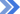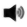## Home Work

Class
Section
Date From
Date To

DateClassAssignment
19 Jul, 2019VII-Springdale Mathematics
Simple equations worksheet19 Jul, 2019VII-Riverdale Mathematics
Simple equations worksheet19 Jul, 2019VII-Daisydale Mathematics
Simple equations worksheet19 Jul, 2019VII-Lovedale Mathematics
Simple equations worksheet19 Jul, 2019VII-Hilltop Mathematics
Simple equations worksheet19 Jul, 2019VII-Rockview Mathematics
Simple equations worksheet"As for me, all I know is that I know nothing. ~ Socrates "